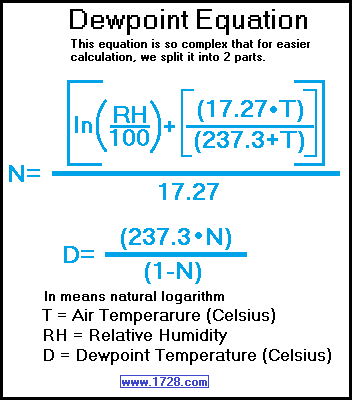Dew Point Calculator

 Do you want to input temperature as  orDew point is defined as the temperature at which dew will form. When the dew point equals the temperature, the relative humudity is 100%. Whenever the relative humidity is less than 100% the dew point is always less than the air temperature. Let's calculate a dew point using the equation above. The air temperature is 20° Celsius and the relative humidity is 50%. What is the dew point? First, we'll solve for 'n'. n = [ln (50 ÷ 100) + (17.27 • 20) ÷ (237.3 + 20)] ÷ 17.27 n = [ln (.5) + 345.4 ÷ 257.3] ÷ 17.27 n = [ -.69314718056 + 1.3424018655] ÷ 17.27 n = 0.6492546849 ÷ 17.27 n = 0.0375943651 Now we put the value of 'n' into the second equation: d = (237.3 • 0.0375943651) ÷ (1 -0.0375943651) d = 8.9211428382 ÷ 0.9624056349 dewpoint = 9.2696286417 Celsius dewpoint = 9.27 Celsius (rounded)

Significant Figures
The default numeric display is 3 significant figures.
For easier readability, numbers that are greater than .001 and less than 1000 are NOT in scientific notation.
You can change the display option by changing the number in the box above.
Entering a zero eliminates all formatting.

"R E T U R N"